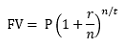Back to contacts

# Compounding Calculator

 Periods Starting Balance Ending Balance Kopējā peļņa Total Gain - - - - -

To calculate the profit earned over the predefined number of periods, use the calculator below.

With a simple input of the starting balance, the number of periods youre compounding the starting balance and the percentage gain per each period. You will the results in a detailed table showing the progress of the investment per each period.

## What is compounding?

Compounding is the action of reinvesting the profits back into the investment in order to increase profits even further, or in other words, getting interest on interest. If youre not reinvesting profits, your investments growth will be linear; when compounding profits, since you will be profiting on the initial investment and also the re-invested capital, growth will become exponential.

## How to calculate compound interest?

You calculate compounded interest using the compounding period profit which can be daily, monthly or annual, and contributing it the number of periods youre interested in.

For example, an annual interest rate of 10% compounded for period of time of 2 years with an initial investment of \$100 would result in \$10 profit for the first year (out of \$100) and \$11 profit for the second year (out of \$110) for a total profit of \$121. If you compare this with a non-compounding investment, it would result in only \$120 since you would get a fixed \$10 profit per each year.

## What is the compounding interest formula?FV = Future Value of your investment

P = Principal or Initial deposit

r = Interest Rate

n = the number of times the investment is compounded in a period

t = number of periods

## Why Compounding Interest is important?

You have a profitable investment? Compounding the profits is the way to go! When your investment is profitable, compounding interest will have a huge impact long term on it.

Albert Einstein once said that compounding is "the most powerful force in the universe" and he was right! The interest you earn on your investment can double and triple your return, even if you have a daily or monthly contribution to your investment.

You might not even know it but if you have a savings account, it is most likely that the interest is compounded at your bank or financial institution.

In the stock market, an account can compound through the reinvestment of dividends while in the forex market, you can reinvest your profits.